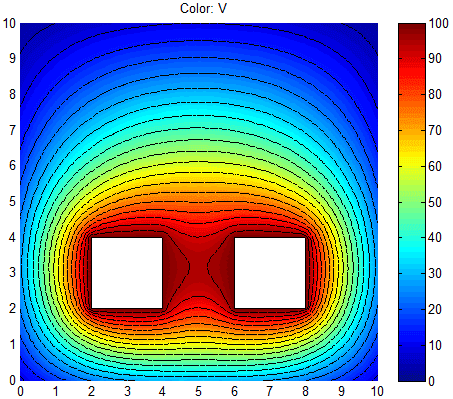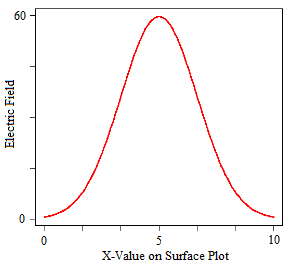# PDETOOL Matlab - Plotting Linear Element?

• MATLAB
Hi,

I've written a script that creates a few rectangles and gives the surface plot in the PDE toolbox.

What I would like to do is to also plot the values for a linear element in the surface plot i.e. for a given value of x or y.

Any help or ideas would be greatly appreciated!

Jay

To add more detail to my problem...

Using the pdetool I created 3 rectangles (using the first method you described), 1 large rectangle (R1) and 2 smaller rectangles (R2 and R3) that are within R1 but not in contact. By applying the formula R1-(R2+R3) and solving it as an electrostatic system in the toolbox I essentially get the cross-sectional surface plot of 2 electrodes (given below).What I would now like to do is for example just look at the values at say y=6 and plot a simple x-y graph which would look something like below:I guess my fundamental problem is not being able to access the information from the surface plot to then manipulate the data. Any insight anyone has into the problem would be greatly appreciated!

Jay

Pythagorean
Gold Member
You have to "get" the "grandchildren" of the figure.

you can use:

child = get(gcf,'Children')
grandchild = get(child,'Children')

then:

X = get(grandchild)

will give you a structure, X, from which you can pull components:

X.Cdata

wil probably then be the data you're looking for, but I'm not sure. Have a look at the fields of X.